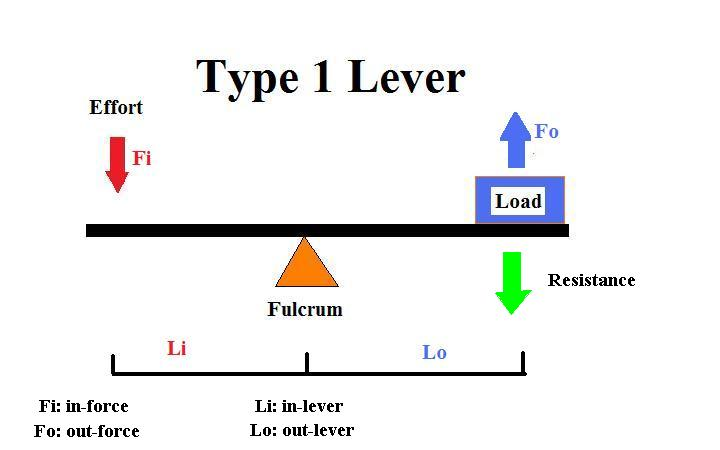What is a lever?

• A lever is one type of simple machine that allows energy to be used in the most advantageous way. Levers can change the 1) direction of the applied force, 2) distance and speed of movement produced by an applied force, 3) effective strength of the applied force.

• A lever is a rigid bar with three parts: the fixed point around which the bar pivots is the fulcrum; the effort arm  (in-lever arm) is the part of the lever to which force is applied; the resistance arm (out-lever arm) is the part that bears the load to be moved.• The effort, or in-force, is applied to the effort arm (in-lever); it is the force put into the machine to move the load.

• The out-force is the force the lever uses to move the load, AFTER the effort force has overcome the resistance of the load (weight). The resistance arm (out-lever) bears the load, so the out-force is produced on this end of the lever to move the load.

• The effort applied to the lever (in-force) must overcome the resistance that the load exerts on the lever (the weight of the load or the force of gravity), in order to produce movement of the load (out-force).

• Mechanical advantage (MA) is simply the ratio of the resistance force to the effort force (MA=Force of resistance/force of effort). *Note: force of resistance is NOT the out-force. To increase the MA, move the fulcrum closer to the load so that you shorten the resistance arm and lengthen the effort arm; this allows you to put the same or less force into the system to move more resistance. By lengthening the effort arm, you are reducing the ratio of the resistance arm to the effort arm (Velocity Ratio), and increasing the ratio of the resistance force to the effort force. The higher the MA, the greater the power of the lever.

• Velocity ratio (VR) is the ratio of the lengths of out-lever to in-lever (length of resistance arm/effort arm). To increase the velocity ratio that the load is moved, increase the resistance arm by moving the fulcrum closer to the effort. The higher the VR, the greater the speed of the lever.

• There are 3 orders of levers that differ only in the relative position of the fulcrum, effort arm, and resistance arm.
1st Order

2nd Order

3rd Order